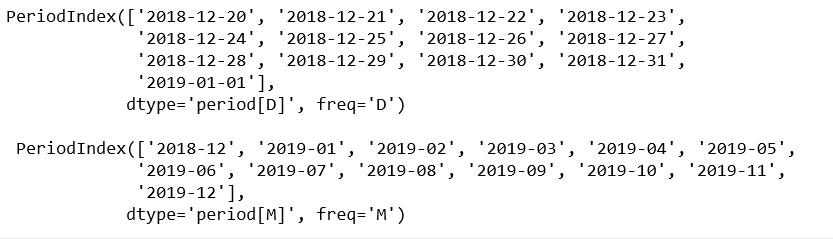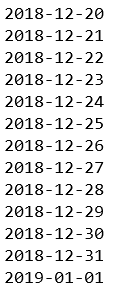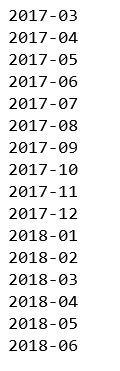Related Articles

# Python | pandas.period_range() method

• Last Updated : 17 Dec, 2018

Python is a great language for doing data analysis, primarily because of the fantastic ecosystem of data-centric python packages. Pandas is one of those packages and makes importing and analyzing data much easier.

`pandas.period_range()` is one of the general functions in Pandas which is used to return a fixed frequency PeriodIndex, with day (calendar) as the default frequency.

Attention geek! Strengthen your foundations with the Python Programming Foundation Course and learn the basics.

To begin with, your interview preparations Enhance your Data Structures concepts with the Python DS Course. And to begin with your Machine Learning Journey, join the Machine Learning - Basic Level Course

Syntax: pandas.to_numeric(arg, errors=’raise’, downcast=None)

Parameters:
start : Left bound for generating periods
end : Right bound for generating periods
periods : Number of periods to generate
freq : Frequency alias
name : Name of the resulting PeriodIndex

Returns: PeriodIndex

Code #1:

 `# importing pandas as pd``import` `pandas as pd`` ` `# period_range with freq = day``per1 ``=` `pd.period_range(start ``=``'2018-12-20'``,``              ``end ``=``'2019-01-01'``, freq ``=``'D'``)`` ` `# period_range with freq = month``per2 ``=` `pd.period_range(start ``=``'2018-12-20'``,``              ``end ``=``'2019-12-01'``, freq ``=``'M'``)`` ` `print``(per1, ``"\n\n"``, per2)`

Output:Code #2:

 `# importing pandas as pd``import` `pandas as pd`` ` `# period_range with freq = day``per1 ``=` `pd.period_range(start ``=``'2018-12-20'``, ``              ``end ``=``'2019-01-01'``, freq ``=``'D'``)`` ` `for` `val ``in` `per1:``    ``print``(val)`

Output:Code #3:

 `# importing pandas as pd``import` `pandas as pd`` ` `# Calling with pd.Period``per ``=` `pd.period_range(start ``=` `pd.Period(``'2017Q1'``, freq ``=``'Q'``),``                ``end ``=` `pd.Period(``'2018Q2'``, freq ``=``'Q'``), freq ``=``'M'``)`` ` `for` `val ``in` `per:``    ``print``(val)`

Output:My Personal Notes arrow_drop_up